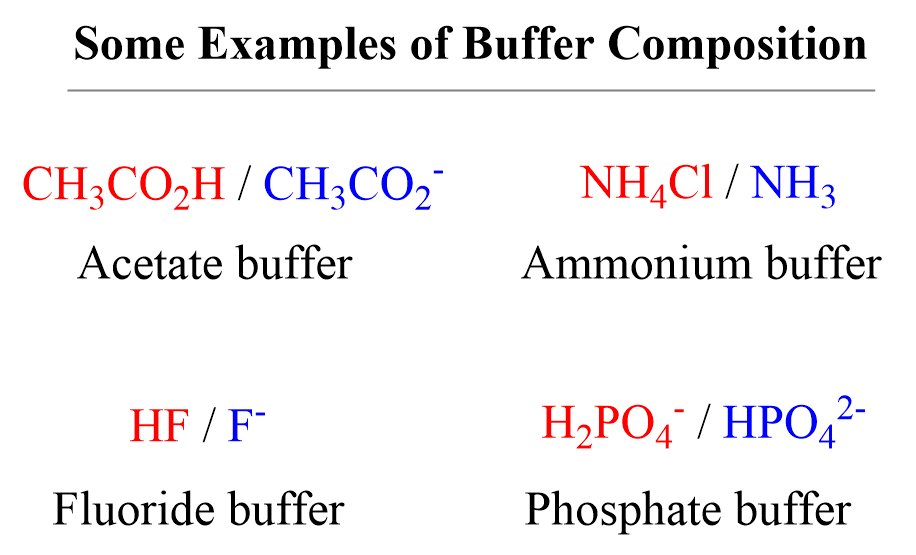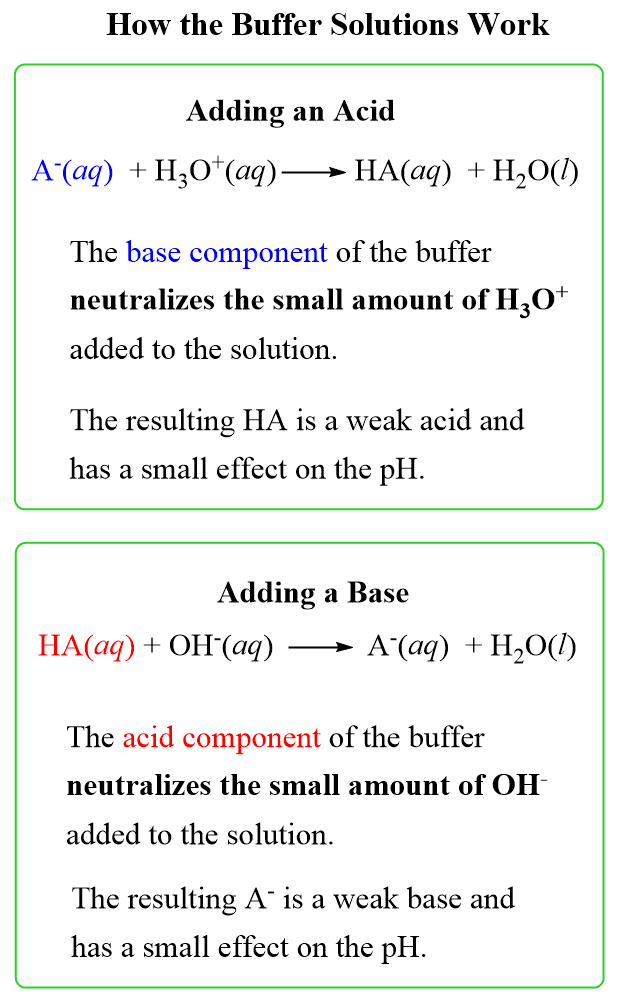## General Chemistry

Most solutions change the pH when an acid or a base is added to them. However, for certain reactions and processes, it is necessary to have a system that keeps the pH constant when a certain amount of an acid or a base is added. To resist a change in pH, a buffer solution is needed. For example, the pH of blood is maintained at about 7.4 while the one of gastric juice in our stomachs stays at around 1.5 even though it could be greatly affected by the food intake.

So, what is a buffer solution?

A buffer is a solution that contains either a weak acid with its salt or a weak base with its salt.The principle behind the mechanism of buffer solutions is the neutralization of the acid or a base added to the solution by reacting it with the basic or the acidic component respectively.

For example, let’s say we add some HCl solution to an acetate buffer. The conjugate base of the acetic acid, sodium acetate CH3CO2Na (can also be shown as acetate ion CH3CO2) will react with and thus neutralizing the excess acid:

HCl(aq) + NaC2H3O2(aq) → HC2H3O2(aq) + NaCl(aq)

On the other hand, if a base such as NaOH is added to the solution, the acetic acid itself will neutralize it and thus preventing the increase of pH:

NaOH(aq) + HC2H3O2(aq) → H2O(l ) + NaC2H3O2(aq)

As a general scheme, the mechanism of buffer solutions can be shown as:There is a limit as to how much acid or a base can be neutralized by the buffer which is called the buffer capacity. For example, if the concentration of acetic acid is 0.10 M, we cannot add an equivalent of 0.20 M NaOH because all the acid will be consumed, and the excess of the base will cause an increase in pH.

This, and the pH of a buffer is what we will cover in the next few posts.

Check Also